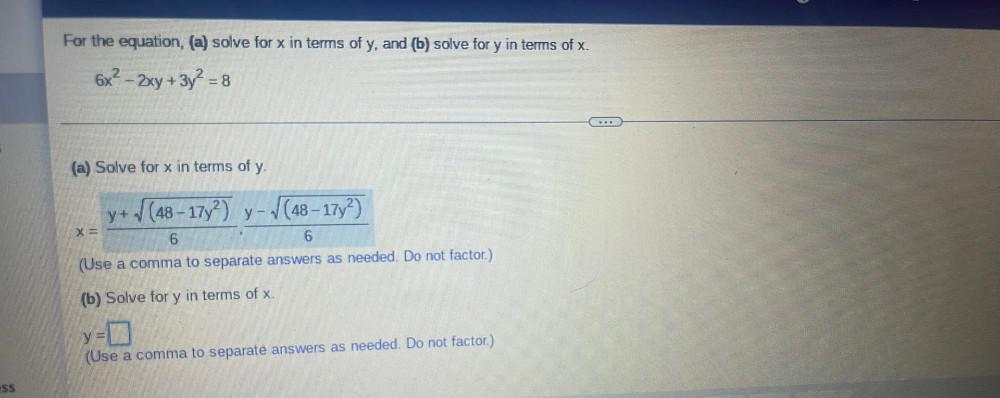Question:

# For the equation, (a) solve for x in terms of y, and (b)

Last updated: 7/26/2022For the equation, (a) solve for x in terms of y, and (b) solve for y in terms of x. 6x² - 2xy + 3y² = 8 (a) Solve for x in terms of y. x = y+√(48 - 17y²)/6, y- √(48 - 17y²)/6 (Use a comma to separate answers as needed. Do not factor.) (b) Solve for y in terms of x. y= ▢ (Use a comma to separate answers as needed. Do not factor)